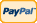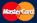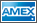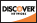Online Store Software Services News About Us My Account Español
•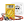I need to measure...
•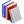I need to calculate...
•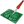Common electronic components repairs and hand soldering techniques
•Electronics, electrical engineering
•Engineering physics
•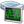Generators
•History events
•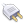Interfaces
•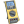Multimeters
•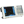Oscilloscopes
•Persona
•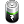Power supplies
•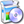Software
•Standards
•Thesaurus
•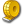Units of measurement

Filter by first letter

# Inductance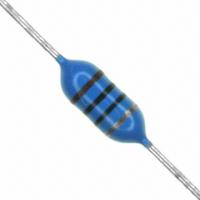In electromagnetism and electronics, inductance is that property of a conductor by which a change in current in the conductor "induces" (creates) a voltage (electromotive force) in both the conductor itself (self-inductance) and any nearby conductors (mutual inductance). This effect derives from two fundamental observations of physics: First, that a steady current creates a steady magnetic field (Oersted's law); second, that a time-varying magnetic field induces voltage in a nearby conductor (Faraday's law of induction). From Lenz's law, in an electric circuit, a changing electric current through a circuit that has inductance induces a proportional voltage which opposes the change in current (self inductance). The varying field in this circuit may also induce an e.m.f. in a neighbouring circuit (mutual inductance).

In the SI system the unit of inductance is the henry.

The term 'inductance' was coined by Oliver Heaviside in February 1886. It is customary to use the symbol L for inductance, in honour of the physicist Heinrich Lenz.

To add inductance to a circuit, electronic components called inductors are used, typically consisting of coils of wire to concentrate the magnetic field and so that the magnetic field is linked into the circuit more than once.

The relationship between the self-inductance L of an electrical circuit in henries, voltage, and current is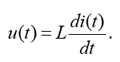where v denotes the voltage in volts and i the current in amperes. The voltage across an inductor is equal to the product of its inductance and the time rate of change of the current through it.

### Inductor Color Coding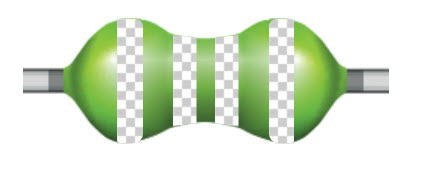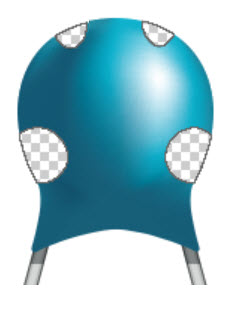SM Inductors or chip inductors use color dots instead of color bands. There are 3 or 4 dots that are read clockwise from the top. The first two dots indicate the significant digits of the inductance value, and the third dot indicates the multiplier.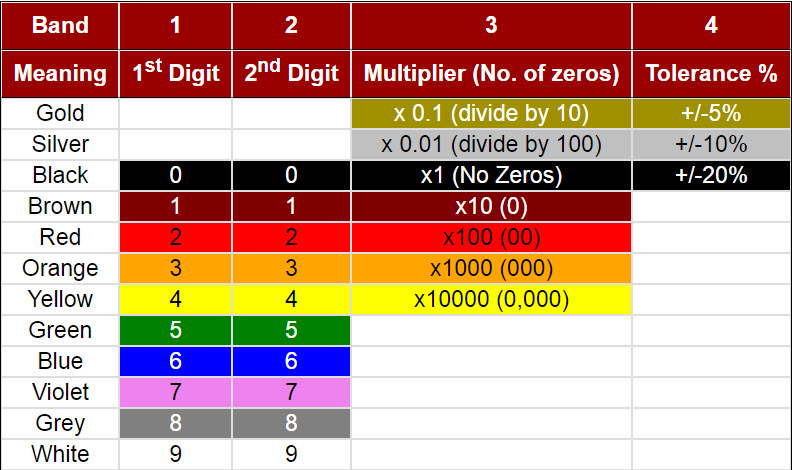To quickly determine inductance and tolerance of an axial inductor use our online Inductor Color Code Calculator. To determine inductance of a SMD (surface mount device) inductor by the markings found on the device please use our online SMD Inductor Code Calculator.

All practical circuits have some inductance, which may provide either beneficial or detrimental effects. In a tuned circuit inductance is used to provide a frequency selective circuit. Practical inductors may be used to provide filtering or energy storage in a system. The inductance of a transmission line is one of the properties that determines its characteristic impedance; balancing the inductance and capacitance of cables is important for distortion-free telegraphy and telephony. The inductance of long power transmission lines limits the AC power that can be sent over them. Sensitive circuits such as microphone and computer network cables may use special cable constructions to limit the mutual inductance between signal circuits.

Back to the list

Measurement History Events
 Yesterday Today Tomorrow
12/08/1880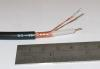Units Converter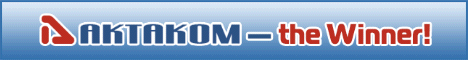Site map|Privacy policy|Terms of Use & Store Policies|How to Buy|Shipping|Payment|© T&M Atlantic, Inc., 2010-2023